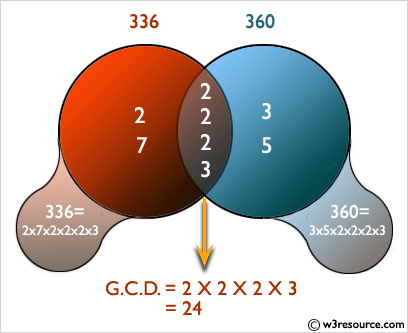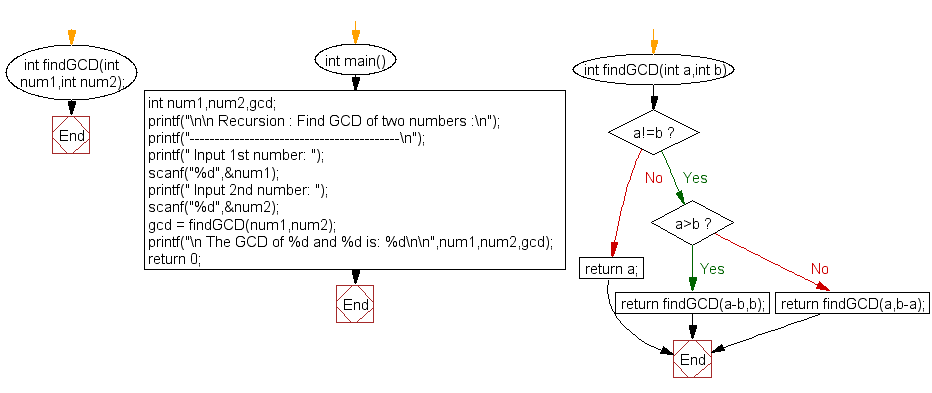﻿ C Program: Find GCD of two numbers - w3resource

# C Exercises: Find GCD of two numbers

## C Recursion : Exercise-7 with Solution

Write a program in C to find the GCD of two numbers using recursion.

Pictorial Presentation:Sample Solution:

C Code:

``````#include<stdio.h>

int findGCD(int num1,int num2);
int main()
{
int num1,num2,gcd;
printf("\n\n Recursion : Find GCD of two numbers :\n");
printf("------------------------------------------\n");
printf(" Input 1st number: ");
scanf("%d",&num1);
printf(" Input 2nd number: ");
scanf("%d",&num2);

gcd = findGCD(num1,num2);
printf("\n The GCD of %d and %d is: %d\n\n",num1,num2,gcd);
return 0;
}

int findGCD(int a,int b)
{
while(a!=b)
{
if(a>b)
return findGCD(a-b,b);
else
return findGCD(a,b-a);
}
return a;
}
```
```

Sample Output:

``` Recursion : Find GCD of two numbers :
------------------------------------------
Input 1st number: 10
Input 2nd number: 50

The GCD of 10 and 50 is: 10
```

Explanation:

```int findGCD(int a,int b)
{
while(a!=b)
{
if(a>b)
return findGCD(a-b,b);
else
return findGCD(a,b-a);
}
return a;
}
```

This function findGCD() takes two integers as parameters (int a, int b) and repeatedly subtracts the smaller number from the larger number until they become equal. When they become equal, the function returns the value of either one of them as the GCD.

Flowchart:C Programming Code Editor:

Have another way to solve this solution? Contribute your code (and comments) through Disqus.

What is the difficulty level of this exercise?

Test your Programming skills with w3resource's quiz.

﻿

## C Programming: Tips of the Day

__FILE__ macro shows full path

```#include <string.h>

#define __FILENAME__ (strrchr(__FILE__, '/') ? strrchr(__FILE__, '/') + 1 : __FILE__)
For Windows use '\\' instead of '/'.
```

Ref : https://bit.ly/3iEWRoT

We are closing our Disqus commenting system for some maintenanace issues. You may write to us at reach[at]yahoo[dot]com or visit us at Facebook